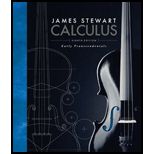# (a) Write the general form of a second-order homogeneous linear differential equation with constant coefficients. (b) Write the auxiliary equation. (c) How do you use the roots of the auxiliary equation to solve the differential equation? Write the form of the solution for each of the three cases that can occur.### Calculus: Early Transcendentals

8th Edition
James Stewart
Publisher: Cengage Learning
ISBN: 9781285741550### Calculus: Early Transcendentals

8th Edition
James Stewart
Publisher: Cengage Learning
ISBN: 9781285741550

#### Solutions

Chapter
Section
Chapter 17, Problem 1RCC
Textbook Problem

## (a) Write the general form of a second-order homogeneous linear differential equation with constant coefficients.(b) Write the auxiliary equation.(c) How do you use the roots of the auxiliary equation to solve the differential equation? Write the form of the solution for each of the three cases that can occur.

Expert Solution

(a)

To determine

To write: The general form of a second-order homogeneous linear differential equation with constant coefficients.

The general form of a second-order homogeneous linear differential equation with constant coefficients is ad2ydx2+bdydx+cy=0_ .

### Explanation of Solution

Formula used:

Consider the second-order linear differential equation as follows.

P(x)d2ydx2+Q(x)dydx+R(x)y=G(x) (1)

Here,

P , Q , R , and G are continuous functions.

If G(x)=0 for all values of x then second-order differential equation is known as homogeneous equation.

Consider the value of G(x) as follows.

G(x)=0

Substitute 0 for G(x) in equation (1),

P(x)d2ydx2+Q(x)dydx+R(x)y=0 (2)

Consider P(x) , Q(x) and R(x) has a constant values represented as a , b and c respectively.

Substitute a for P(x) , b for Q(x) and c for R(x) in equation (2),

Thus, the general form of a second-order homogeneous linear differential equation with constant coefficients is ad2ydx2+bdydx+cy=0_ .

Expert Solution

(b)

To determine

To write: The auxiliary equation.

The auxiliary equation of the second-order differential equation is ar2+br+c=0_ .

### Explanation of Solution

Modify equation (3) as follows.

ay+by+cy=0 (4)

In equation (4), function y such that a constant times its second derivative (y) plus second constant times its second derivative (y) plus a third constant times y is equal to 0 .

Consider a exponential function for y as follows.

y=erx

Differentiate y with respect to x .

y=rerx

Differentiate y with respect to x .

y=r2erx

Substitute r2erx for y , rerx for y and erx for y in equation (4),

ar2erx+rberx+cerx=0

erx(ar2+br+c)=0 (5)

Since, the value of erx is never 0 , erx becomes the solution for y with r is a root of the equation.

ar2+br+c=0 (6)

Equation (6) is known as characteristic equation or auxiliary equation of the second-order differential equation ay+by+cy=0 .

Thus, the auxiliary equation of the second-order differential equation is ar2+br+c=0_ .

Expert Solution

(c)

To determine

To explain: The use of roots of the auxiliary equation to solve the differential equation and write the form of the solution for each of the three cases.

The use of roots of the auxiliary equation to solve the differential equation is explained.

The form of the solution for each of the three cases is written.

### Explanation of Solution

Formula used:

Consider the second-order differential equation as follows.

ax2+bx+c=0

Write the expression for quadratic formula.

x=b±b24ac2a

x consists of two roots represented by x1 and x2 as follows.

x1=b+b24ac2a (7)

x2=bb24ac2a (8)

Modify equation (7) as follows.

r1=b+b24ac2a

Modify equation (8) as follows.

r2=bb24ac2a

r1 and r2 are the roots of auxiliary equation ar2+br+c=0 .

Thus, the use of roots of the auxiliary equation to solve the differential equation is explained.

Three different cases are obtained depending upon the term b24ac present in the roots of auxiliary equation.

Case I:

Consider the value of b24ac>0 .

In this case, the roots of auxiliary equation are real and distinct. So two linear independent solutions are occurs such as y1=er1x and y2=er2x .

Write the expression for general solution.

y=c1y1+c2y2 (9)

Here,

c1 and c2 are constants.

Substitute er1x for y1 and er2x for y2 ,

y=c1er1x+c2er2x

Case II:

Consider the value of b24ac=0 .

In this case, the roots of auxiliary equation are real and equal.

Consider r be the common roots for r1 and r2 . So the solutions are occurs such as y1=erx and y2=xerx .

Substitute erx for y1 and xerx for y2 in equation (9),

y=c1erx+xc2erx

Case III:

Consider the value of b24ac<0 .

In this case, the roots of auxiliary equation are complex numbers.

Consider r1 and r2 are represented as follows.

r1=α+iβr2=αiβ

Write the expression for general solution with complex roots.

y=eαx(c1cosβx+c2sinβx)

Thus, the form of the solution for each of the three cases is written.

### Want to see more full solutions like this?

Subscribe now to access step-by-step solutions to millions of textbook problems written by subject matter experts!

Get Solutions

### Want to see more full solutions like this?

Subscribe now to access step-by-step solutions to millions of textbook problems written by subject matter experts!

Get Solutions